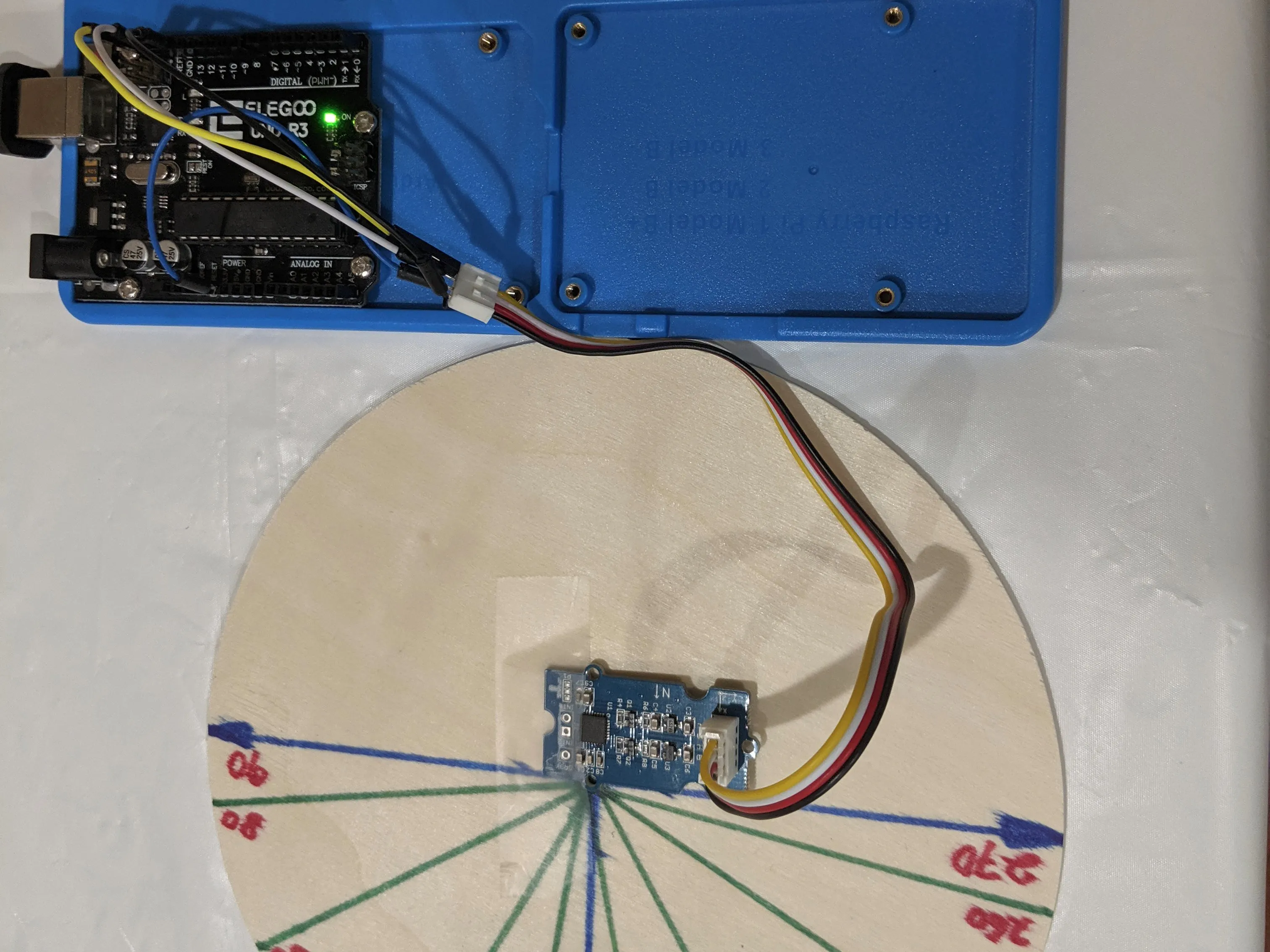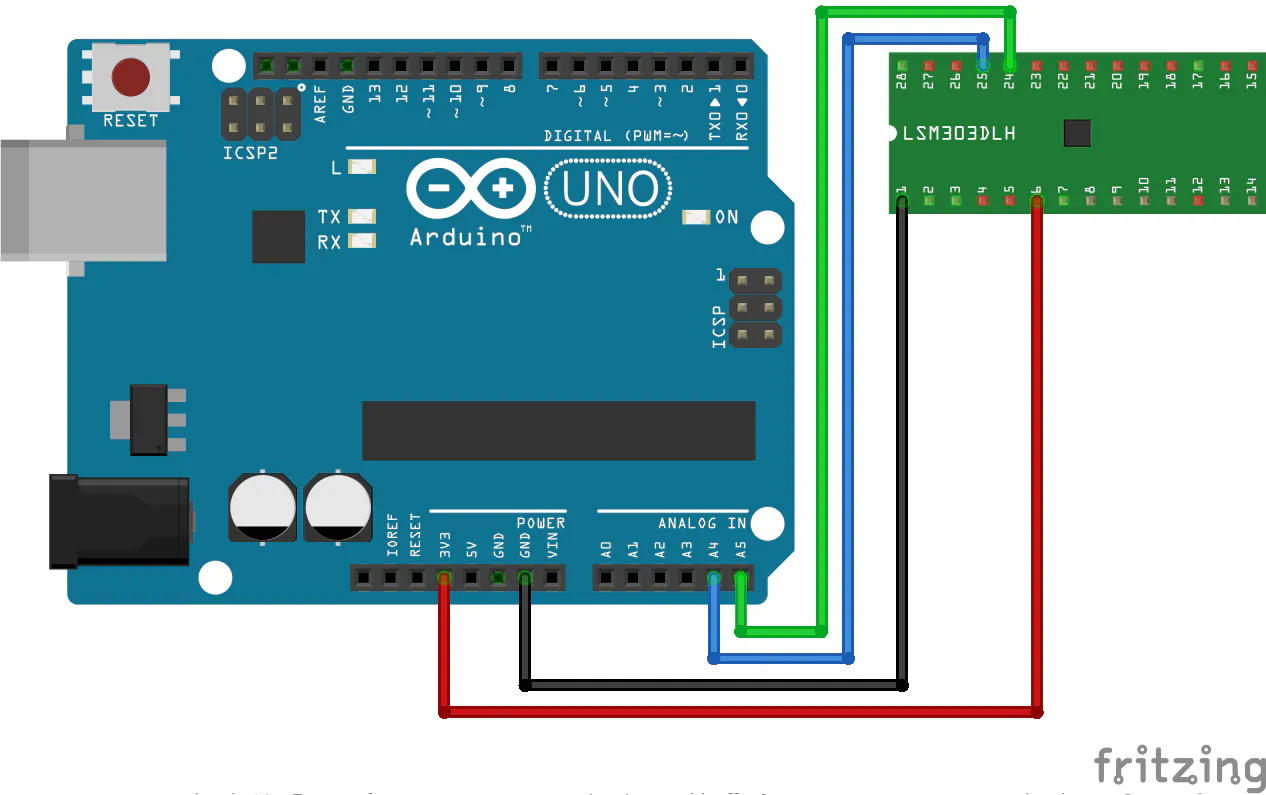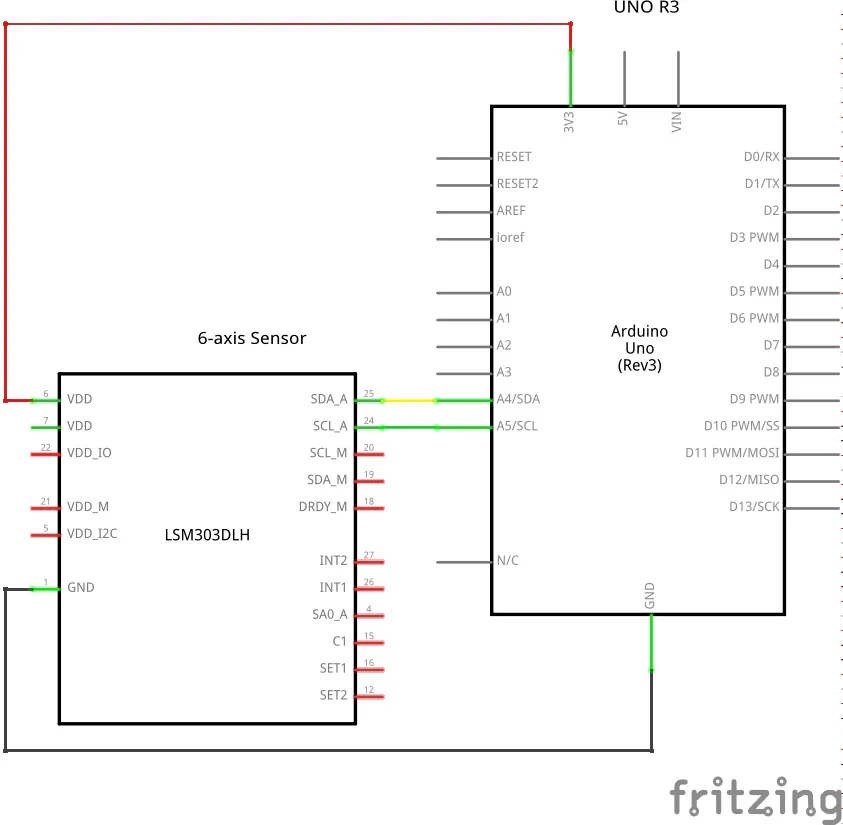Use a 6-axis sensor (compass + accelerometer) to refine your heading estimates as you weave through in three-dimensional space.

BeginnerProtip15 minutes899## Things used in this project

### Hardware components

 Elegoo Arduino UNO R3
×1
 Elegoo DuPont connecting wires
×4
 Seeed Grove - 6-Axis Accelerometer&Compass V2.0
×1

### Software apps and online servicesArduino IDE

## Custom parts and enclosures

### Assembly diagram

Direct connection example## Schematics

### Basic schematic diagram

No breadboard needed for basic sanity test.## Code

### LSM303D-05.ino

C/C++
Basic test exercise
```/* LSM303DLM Example Code base on LSM303DLH example code by Jim Lindblom SparkFun Electronics

date: 9/6/11

Modified by:Frankie.Chu

Summary:
Show how to calculate level and tilt-compensated heading using
the snazzy LSM303DLH 3-axis magnetometer/3-axis accelerometer.

Firmware:
You can set the accelerometer's full-scale range by setting
the SCALE constant to either 2, 4, or 8. This value is used
in the initLSM303() function. For the most part, all other
registers in the LSM303 will be at their default value.

Use the LSM303_write() and LSM303_read() functions to write
to and read from the LSM303's internal registers.

Use getLSM303_accel() and getLSM303_mag() to get the acceleration
and magneto values from the LSM303. You'll need to pass each of
those functions an array, where the data will be stored upon
return from the void.

A float between 0 and 360 is returned. You need to pass it a
array with magneto values.

A float between 0 and 360 degrees is returned. You need
to pass this function both a magneto and acceleration array.

Headings are calculated as specified in AN3192:
http://www.sparkfun.com/datasheets/Sensors/Magneto/Tilt%20Compensated%20Compass.pdf

Hardware:
I'm using SparkFun's LSM303 breakout. Only power and the two
I2C lines are connected:
LSM303 Breakout ---------- Arduino
Vin                   5V
GND                   GND
SDA                   A4
SCL                   A5
*/
#include <Wire.h>
#include <math.h>

#define ACCELE_SCALE 2  // accelerometer full-scale, should be 2, 4, or 8

#define LSM303_MAG  0x1E  // assuming SA0 grounded
#define LSM303_ACC  0x18  // assuming SA0 grounded

#define X 0
#define Y 1
#define Z 2

/* LSM303 Register definitions */
#define CTRL_REG1_A 0x20
#define CTRL_REG2_A 0x21
#define CTRL_REG3_A 0x22
#define CTRL_REG4_A 0x23
#define CTRL_REG5_A 0x24
#define HP_FILTER_RESET_A 0x25
#define REFERENCE_A 0x26
#define STATUS_REG_A 0x27
#define OUT_X_L_A 0x28
#define OUT_X_H_A 0x29
#define OUT_Y_L_A 0x2A
#define OUT_Y_H_A 0x2B
#define OUT_Z_L_A 0x2C
#define OUT_Z_H_A 0x2D
#define INT1_CFG_A 0x30
#define INT1_SOURCE_A 0x31
#define INT1_THS_A 0x32
#define INT1_DURATION_A 0x33
#define CRA_REG_M 0x00
#define CRB_REG_M 0x01//refer to the Table 58 of the datasheet of LSM303DLM
#define MAG_SCALE_1_3 0x20//full-scale is +/-1.3Gauss
#define MAG_SCALE_1_9 0x40//+/-1.9Gauss
#define MAG_SCALE_2_5 0x60//+/-2.5Gauss
#define MAG_SCALE_4_0 0x80//+/-4.0Gauss
#define MAG_SCALE_4_7 0xa0//+/-4.7Gauss
#define MAG_SCALE_5_6 0xc0//+/-5.6Gauss
#define MAG_SCALE_8_1 0xe0//+/-8.1Gauss
#define MR_REG_M 0x02
#define OUT_X_H_M 0x03
#define OUT_X_L_M 0x04
#define OUT_Y_H_M 0x07
#define OUT_Y_L_M 0x08
#define OUT_Z_H_M 0x05
#define OUT_Z_L_M 0x06
#define SR_REG_M 0x09
#define IRA_REG_M 0x0A
#define IRB_REG_M 0x0B
#define IRC_REG_M 0x0C

/* Global variables */
int16_t accel;  // we'll store the raw acceleration values here
int16_t mag;  // raw magnetometer values stored here
float realAccel;  // calculated acceleration values here

void setup()
{
Serial.begin(115200);  // Serial is used for debugging
Wire.begin();  // Start up I2C, required for LSM303 communication
initLSM303(ACCELE_SCALE);  // Initialize the LSM303, using a SCALE full-scale range
}

void loop()
{
//Serial.println("**************");
getLSM303_accel(accel);  // get the acceleration values and store them in the accel array
getLSM303_mag(mag);  // get the magnetometer values, store them in mag
//printValues(mag, accel);  // print the raw accel and mag values, good debugging
//Serial.print("Acc., g: X, Y, Z: ");
for (int i=0; i<3; i++)
{
realAccel[i] = accel[i] / pow(2, 15) * ACCELE_SCALE;  // calculate real acceleration values, in units of g
//Serial.print(realAccel[i]);
//Serial.print(" ");
}
Serial.println();
/* print both the level, and tilt-compensated headings below to compare */
//Serial.print("CW (Nm & X): ");
Serial.print(getHeading(mag), 0); // this only works if the sensor is level
//Serial.print("\u00B0; CW (Nm & P:");
//Serial.print(getTiltHeading(mag, realAccel), 0);  // see how awesome tilt compensation is?!
//Serial.println("\u00B0");
delay(100);  // delay for serial readability
}

void initLSM303(int fs)
{
LSM303_write(0x27, CTRL_REG1_A);  // 0x27 = normal power mode, all accel axes on
if ((fs==8)||(fs==4))
LSM303_write((0x00 | (fs-fs/2-1)<<4), CTRL_REG4_A);  // set full-scale
else
LSM303_write(0x00, CTRL_REG4_A);
LSM303_write(0x14, CRA_REG_M);  // 0x14 = mag 30Hz output rate
LSM303_write(MAG_SCALE_1_3, CRB_REG_M); //magnetic scale = +/-1.3Gauss
LSM303_write(0x00, MR_REG_M);  // 0x00 = continouous conversion mode
}

void printValues(int16_t * magArray, int16_t * accelArray)
{
/* print out mag and accel arrays all pretty-like */
Serial.print(accelArray[X], DEC);

Serial.print("\t");
Serial.print(accelArray[Y], DEC);
Serial.print("\t");
Serial.print(accelArray[Z], DEC);
Serial.print("\t\t");

Serial.print(magArray[X], DEC);
Serial.print("\t");
Serial.print(magArray[Y], DEC);
Serial.print("\t");
Serial.print(magArray[Z], DEC);
Serial.println();
}
{
// see section 1.2 in app note AN3192
float heading = 180*atan2(magValue[Y], magValue[X])/PI;  // assume pitch, roll are 0

}

float getTiltHeading(int16_t * magValue, float * accelValue)
{
// see appendix A in app note AN3192
float pitch = asin(-accelValue[X]);
float roll = asin(accelValue[Y]/cos(pitch));

float xh = magValue[X] * cos(pitch) + magValue[Z] * sin(pitch);
float yh = magValue[X] * sin(roll) * sin(pitch) + magValue[Y] * cos(roll) - magValue[Z] * sin(roll) * cos(pitch);
float zh = -magValue[X] * cos(roll) * sin(pitch) + magValue[Y] * sin(roll) + magValue[Z] * cos(roll) * cos(pitch);
float heading = 180 * atan2(yh, xh)/PI;

if (yh >= 0)    return heading;
}

void getLSM303_mag(int16_t * rawValues)
{
Wire.beginTransmission(LSM303_MAG);
Wire.write(OUT_X_H_M);
Wire.endTransmission();
Wire.requestFrom(LSM303_MAG, 6);
for (int i=0; i<3; i++)
int temp;
temp = rawValues[Y];
rawValues[Y] = rawValues[Z];
rawValues[Z] = temp;
}

void getLSM303_accel(int16_t * rawValues)
{
// had to swap those to right the data with the proper axis
}

{
byte temp;

Wire.beginTransmission(LSM303_ACC);
else
Wire.beginTransmission(LSM303_MAG);

Wire.requestFrom(LSM303_ACC, 1);
else
Wire.requestFrom(LSM303_MAG, 1);
while(!Wire.available())
;
Wire.endTransmission();

return temp;
}

{
Wire.beginTransmission(LSM303_ACC);
else
Wire.beginTransmission(LSM303_MAG);

Wire.write(data);
Wire.endTransmission();
}
```

### LSM303D-01.ino

C/C++
``` // --------------------------------------
// i2c_scanner
//
// Version 1
//    This program (or code that looks like it)
//    can be found in many places.
//    For example on the Arduino.cc forum.
//    The original author is not know.
// Version 2, Juni 2012, Using Arduino 1.0.1
//     Adapted to be as simple as possible by Arduino.cc user Krodal
// Version 3, Feb 26  2013
//    V3 by louarnold
// Version 4, March 3, 2013, Using Arduino 1.0.3
//    by Arduino.cc user Krodal.
//    Changes by louarnold removed.
//    Scanning addresses changed from 0...127 to 1...119,
//    according to the i2c scanner by Nick Gammon
//    https://www.gammon.com.au/forum/?id=10896
// Version 5, March 28, 2013
//    As version 4, but address scans now to 127.
//    A sensor seems to use address 120.
// Version 6, November 27, 2015.
//    Added waiting for the Leonardo serial communication.
//
//
// This sketch tests the standard 7-bit addresses
// Devices with higher bit address might not be seen properly.
//

#include <Wire.h>

void setup()
{
Wire.begin();

Serial.begin(115200);
while (!Serial);             // Leonardo: wait for serial monitor
Serial.println("\nI2C Scanner");
}

void loop()
{
int nDevices;

Serial.println("Scanning...");

nDevices = 0;
{
// The i2c_scanner uses the return value of
// the Write.endTransmisstion to see if
// a device did acknowledge to the address.
error = Wire.endTransmission();

if (error == 0)
{
Serial.print("I2C device found at address 0x");
Serial.print("0");
Serial.print(", 0d");
Serial.print(", 0o");
Serial.print(", 0b");

nDevices++;
}
else if (error==4)
{
Serial.print("0");
}
}
if (nDevices == 0)
Serial.println("No I2C devices found\n");
else
Serial.println("done\n");

delay(5000);           // wait 5 seconds for next scan
}
```

## Credits

### Matha Goram

22 projects • 16 followers
Working with discrete electronic components for a very long time but still suffering from the occasional dry soldering results.
Thanks to Frankie Chu and Jim Lindblom.